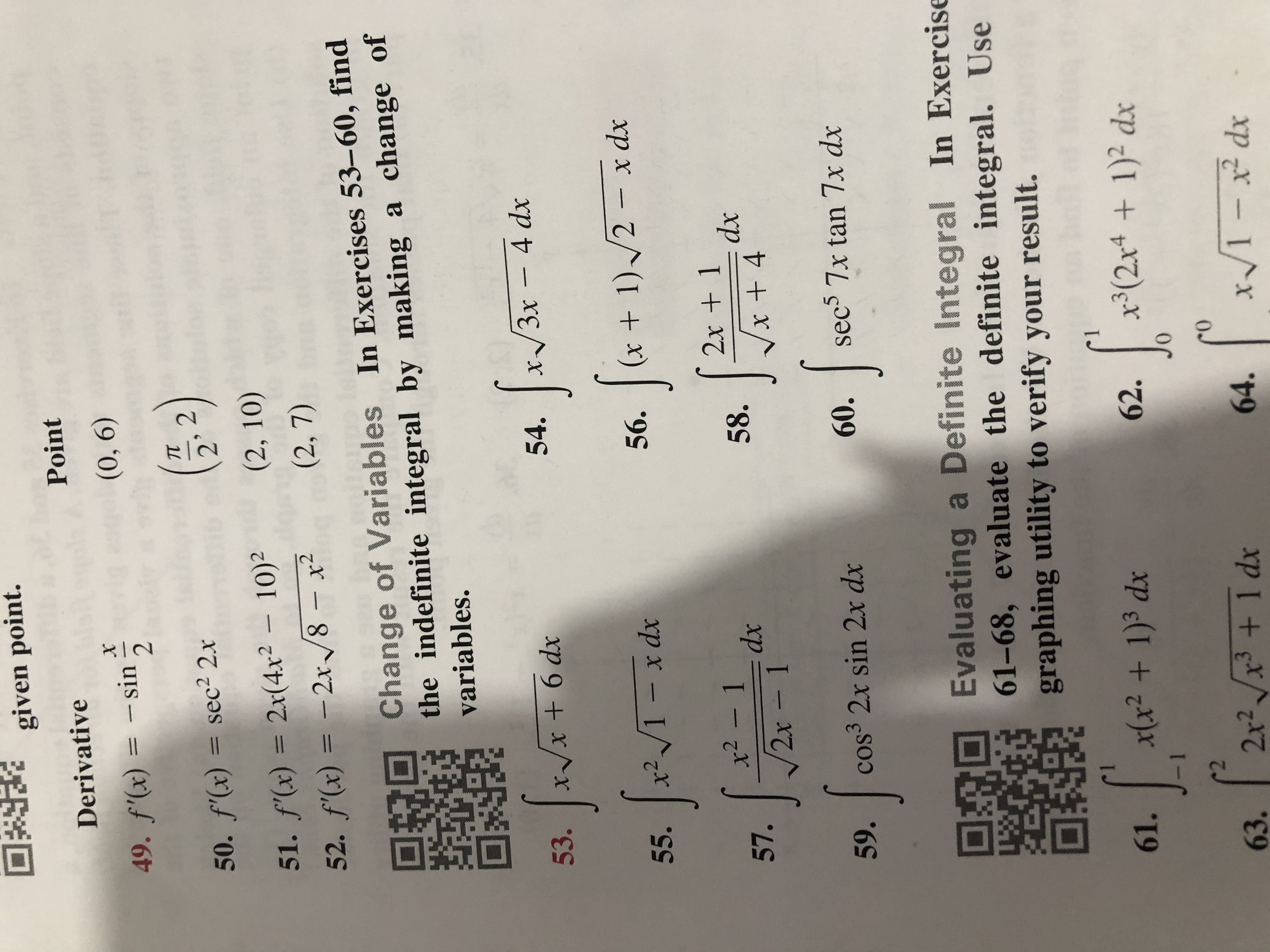# given point.PointDerivative(0, 6)49. f(x) -sin(-2)50. f(x) = sec2 2x51. f'(x) 2x(4x2 10)252. f'(x) =-2x/8- x2(2, 10)(2, 7)Change of Variables In Exercises 53-60, findthe indefinite integral by making a change ofvariables.3x - 4 dx54.x +6 dx53.1)/2- x dx(xV1 - x dx56.55.2x 1dxx- 1dx2x - 158.57.sec5 7x tan 7x dxcos3 2x sin 2x dx59.60.Evaluating a Definite Integral61-68, evaluate the definite integral. Usegraphing utility to verify your result.In ExercisexX2 + 1)3 dx61.t(2x4 1)2 dx62.2x3 +1 dx63.x/1- x2 dx64.

Question
14 views

55help_outlineImage Transcriptionclosegiven point. Point Derivative (0, 6) 49. f(x) -sin (-2) 50. f(x) = sec2 2x 51. f'(x) 2x(4x2 10)2 52. f'(x) =-2x/8- x2 (2, 10) (2, 7) Change of Variables In Exercises 53-60, find the indefinite integral by making a change of variables. 3x - 4 dx 54. x +6 dx 53. 1)/2- x dx (x V1 - x dx 56. 55. 2x 1 dx x- 1 dx 2x - 1 58. 57. sec5 7x tan 7x dx cos3 2x sin 2x dx 59. 60. Evaluating a Definite Integral 61-68, evaluate the definite integral. Use graphing utility to verify your result. In Exercise xX2 + 1)3 dx 61. t(2x4 1)2 dx 62. 2 x3 +1 dx 63. x/1- x2 dx 64. fullscreen
check_circle

Step 1

Given,

Step 2

Let t = 1 – x

Differentiate with respect to x, it gives

Step 3

Therefore...

### Want to see the full answer?

See Solution

#### Want to see this answer and more?

Solutions are written by subject experts who are available 24/7. Questions are typically answered within 1 hour.*

See Solution
*Response times may vary by subject and question.
Tagged in

### Integration